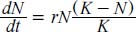NextPrevious

# What is a logistic equation?

A logistic equation (resulting in a curve on a graph) represents the exponential increase in numbers of a species until it reaches the carrying capacity in its specific environment. This carrying capacity, usually referred to by the letter K, is the maximum population size that can be regularly sustained by an environment. Change the environment and K changes, for example, by such events as adding a predator, removing a competitor, or adding a parasite. The notation that follows (in the form of a differential equation) represents a rate of population increase that is limited by interspecific competition (see above):in which N is the population size, t is time, K is the carrying capacity, and r is the intrinsic rate of increase.

Close

This is a web preview of the "The Handy Math Answer Book" app. Many features only work on your mobile device. If you like what you see, we hope you will consider buying. Get the App